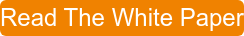Bob Bickler

When it comes to isolating a compound from a mixture at maximum purity there are many options available through flash column chromatography. In previous posts I have addressed using smaller particle media, higher surface area media, and step gradients to achieve this goal.

In this post I will discuss how stacking columns in series may help improve separation quality.

Recently, I was asked if there is any real benefit to stacking columns.  Many chemists have the perception that the column length to diameter ratio (L/D ratio) plays a role in separating power.

A while ago I wrote a couple of posts asking the question “Does size matter in flash chromatography” where I discussed both media physical properties in one and flash cartridge length vs. diameter in another.

Indeed, chromatographic theory tells us a longer column contains more theoretical plates and more theoretical plates provide a better separation, Equation 1.

N = 5.54(tr/W½)²                                        Equation 1

Where N = plates per column

tr = compound retention

W½ = compound peak width at 50% of its height

Plate count, or efficiency as it is also known, increases with column length only if peak width increases at a slower rate than compound retention increases, which is not always the case.

In flash chromatography theoretical plates do matter, of course, but only to the extent that you have sufficient selectivity for the separation and use the correct flow rate for the selected column. That being the case, then the loading amount and separation quality should be more about having the correct sample size per gram of media than column dimensions.

To evaluate this theorem, I conducted a test where I compared a 30 gram flash column (Biotage® ZIP 30 gram) with a 3 x 10 gram column stack (Biotage ZIP 10 gram), all with the same silica, Table 1. I compared these at equal flow rate and again at equal linear velocity to see where there is a performance difference, if any.

 30 gram column 10 gram column 3 x 10 gram stack 3 x 10 gram stack Length (cm) 11.6 6.9 20.7 20.7 Diameter (cm) 2.7 2 2 2 L/D ratio 4.3 3.45 10.35 10.35 Flow rate (mL/min) 60 60 34 Linear velocity (cm/min) 15.5 27.6 15.6First, I used a 30 gram column and purified a 5-component mix targeting the fourth eluting compound. To determine sample load I used my flash system’s automated TLC to linear gradient capability based on a TLC solvent system of 20% EtOAc in hexane. The system suggested my load should be ~250 mg. In Figure 1 you can see the results of this prediction and the resulting chromatography.

Figure 1. Linear gradient using a 30 gram silica column with a 250 mg sample load at a flow rate of 60 mL/min.  The separation quality is good though baseline resolution was not achieved between peaks 4 and 5 (peak 4 in pink is the target compound).

Second, I repeated the same method but replaced the 30 gram column with three 10 gram columns in series. I maintained both flow rate and sample size, Figure 2.Figure 2. Linear gradient purification using three 10 gram silica columns in series using the same 60 mL/min flow rate used with the 30 gram column. The peaks are slightly more narrow but compound resolution (peaks 4 and 5) has not improved much.

Last, I used another series of three 10 gram columns using the same gradient profile and load but running at a flow rate at which I achieved a similar linear velocity to the 30 gram cartridge (~15.5 cm/min), Figure 3.Figure 3. Linear gradient using three 10 gram silica columns at the same linear velocity as the 30 gram cartridge (~15.5 cm/min). At this flow we see resolution improvements due to reduced band broadening.

The results show that there is minor separation enhancement for my target compound (fourth peak) and the last compound when the flow rate was maintained (60 mL/min). The peaks do appear a bit more narrow with the column stack.

When the flow rate was reduced to equalize linear velocity to that used with the 30 gram column the impact of the longer column length is seen with sharper peaks and improved resolution.

So, what does this tell us?   It tells us that column stacking really only improves your separation if you adjust the flow linear velocity and that L/D ratio alone is not an important factor in separation performance as borne out in the TLC analysis of peak 4, Figure 4.Figure 4. TLC analysis of 30 g vs. stacked 10 g columns. Far left - fractions 9, 10, and 11 for the 30 gram column fractions. Far right - fractions 9, 10, and 11 for the 3 x 10 g column stack at 60 mL/min. In both cases fraction 11 shows an impurity. Center - because of the improved separation using the column stack at lower flow only two fractions, 9 and 10, were needed to collect peak 4. The TLC analysis of these fractions does not show the presence of any other compound.

Have you tried stacking columns to improve your tough separations?  If so, did it improve your results?